Perpendicular Lines

Chapter 5 Class 6 Understanding Elementary Shapes
Concept wise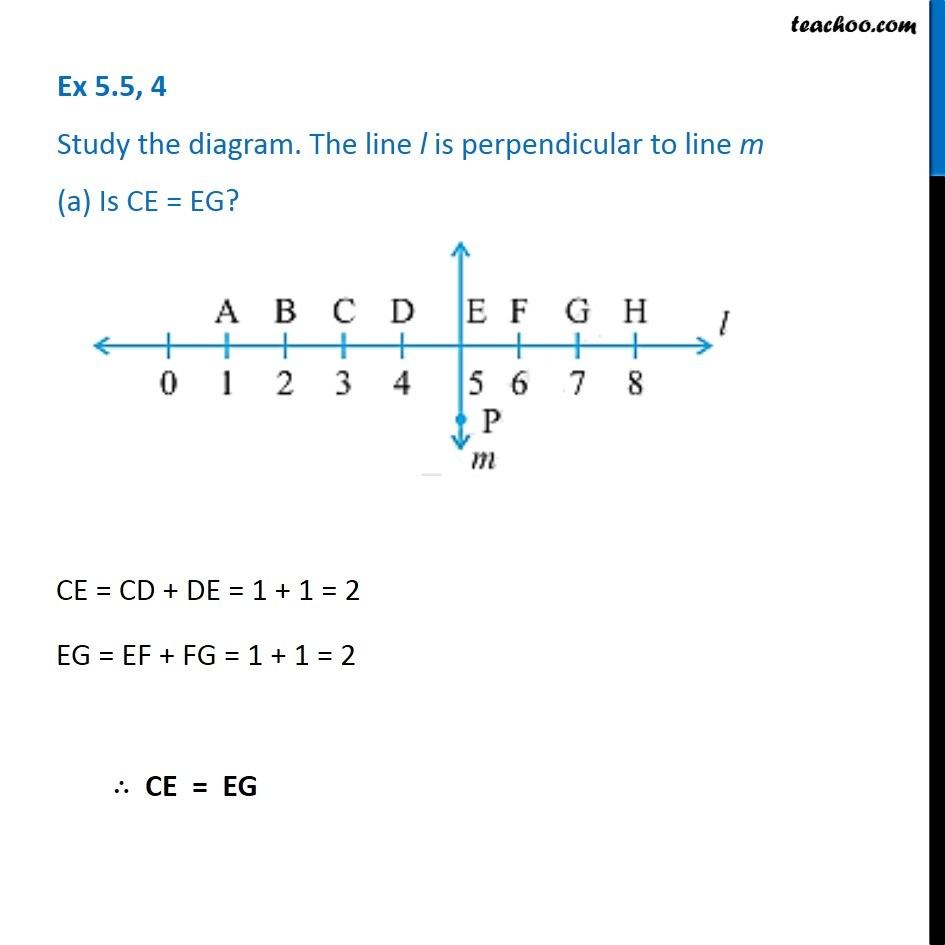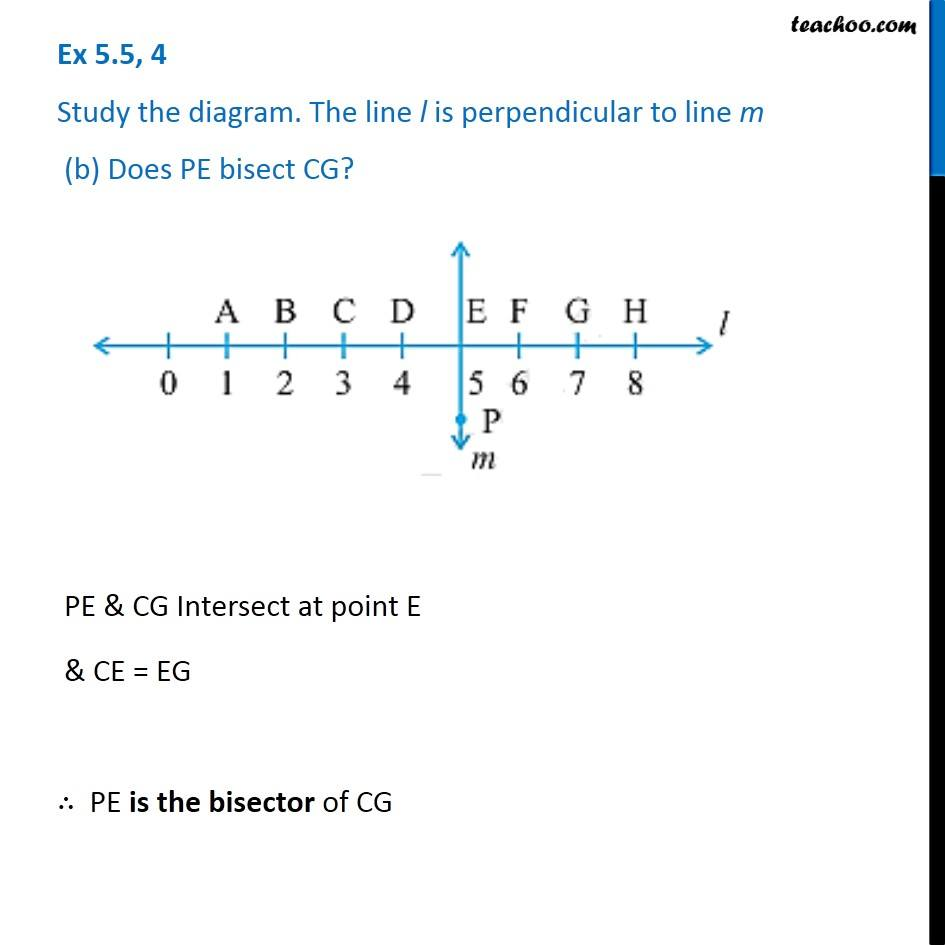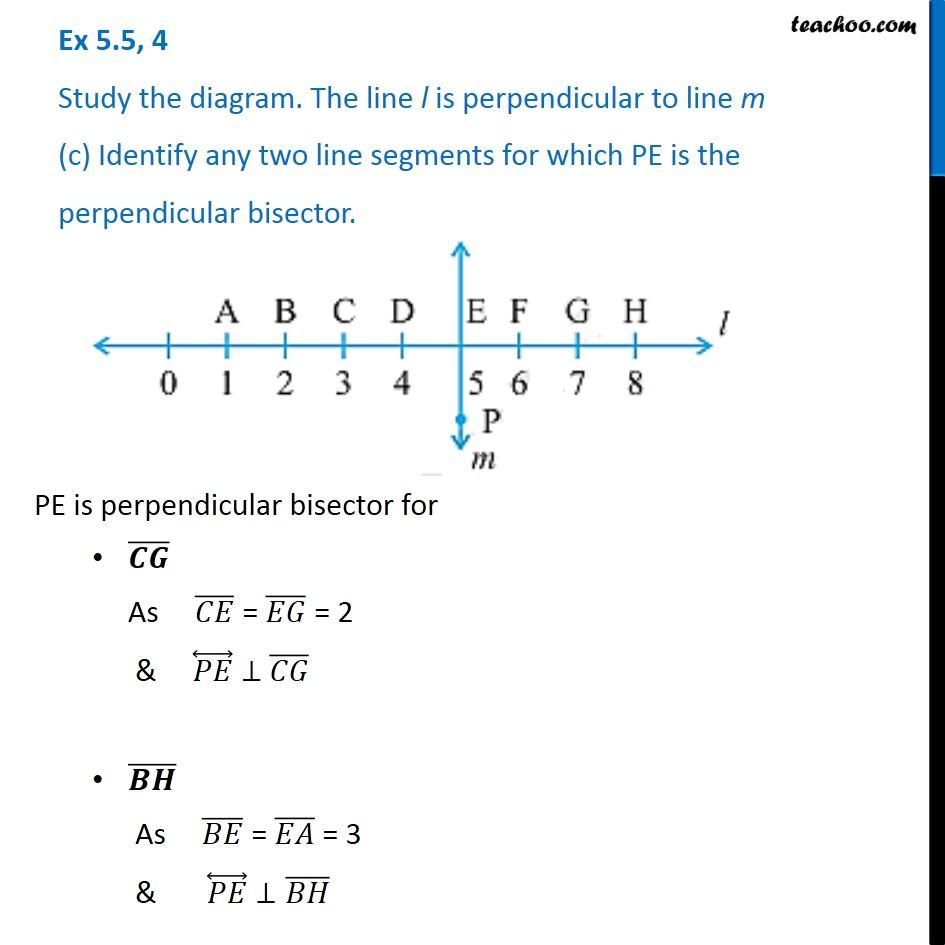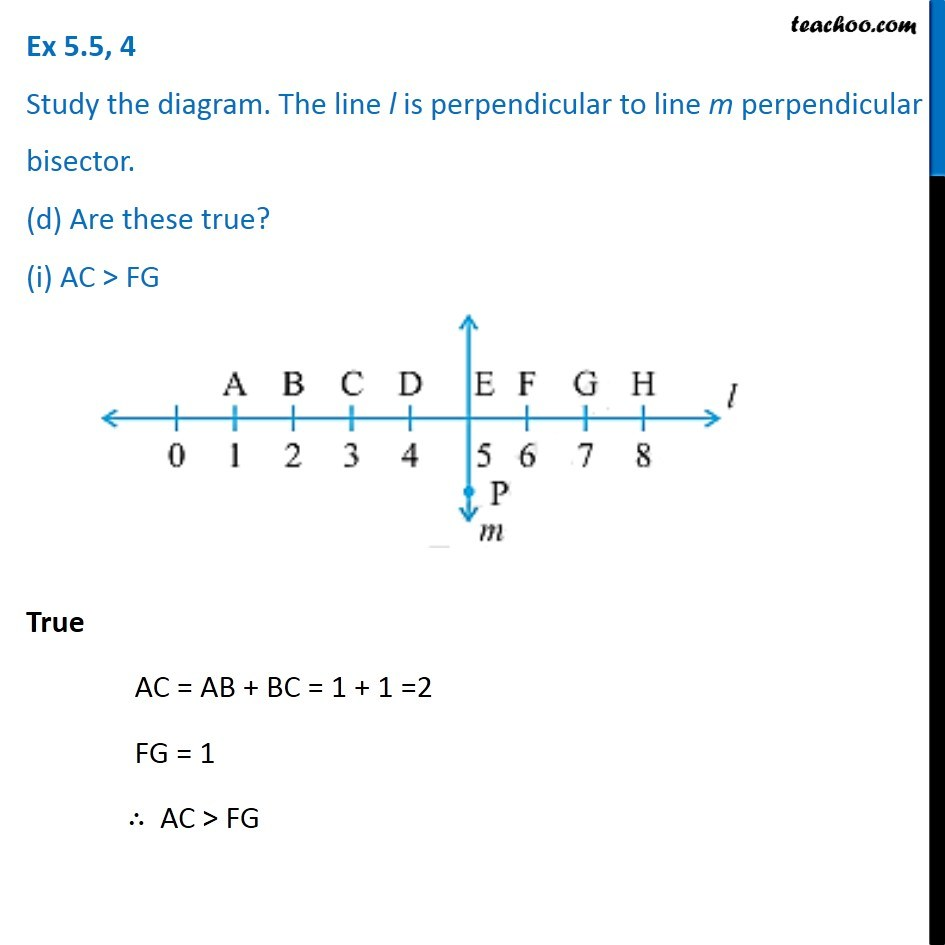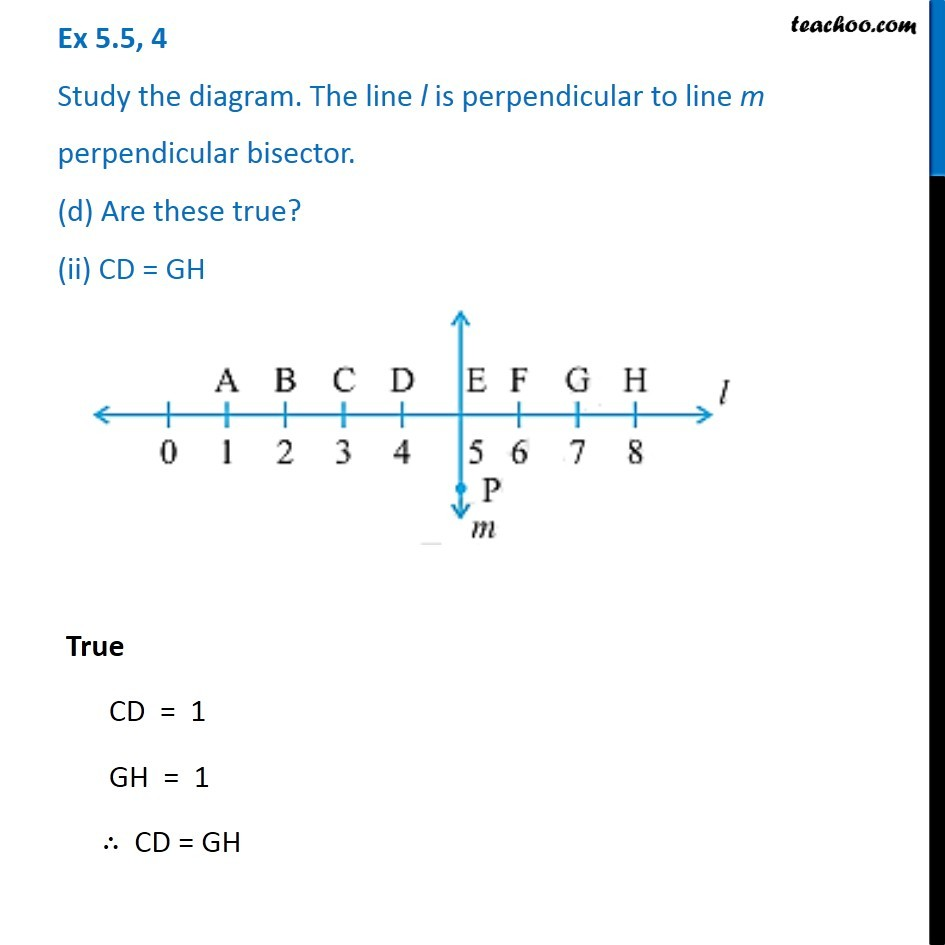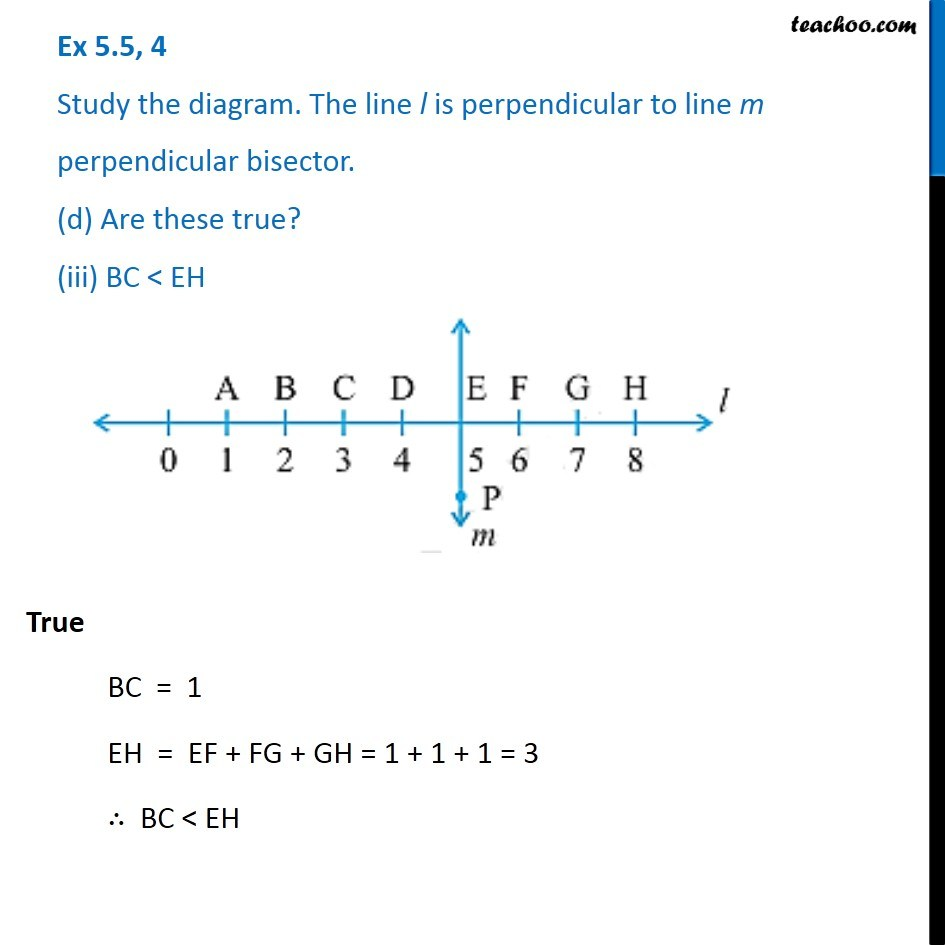Learn in your speed, with individual attention - Teachoo Maths 1-on-1 Class

### Transcript

Ex 5.5, 4 Study the diagram. The line l is perpendicular to line m (a) Is CE = EG? CE = CD + DE = 1 + 1 = 2 EG = EF + FG = 1 + 1 = 2 ∴ CE = EG Ex 5.5, 4 Study the diagram. The line l is perpendicular to line m (b) Does PE bisect CG? PE & CG Intersect at point E & CE = EG ∴ PE is the bisector of CG Ex 5.5, 4 Study the diagram. The line l is perpendicular to line m (c) Identify any two line segments for which PE is the perpendicular bisector. PE is perpendicular bisector for (𝑪𝑮) ̅ As (𝐶𝐸) ̅ = (𝐸𝐺) ̅ = 2 & (𝑃𝐸) ⃡ ⊥ (𝐶𝐺) ̅ (𝑩𝑯) ̅ As (𝐵𝐸) ̅ = (𝐸𝐴) ̅ = 3 & (𝑃𝐸) ⃡ ⊥(𝐵𝐻) ̅ Ex 5.5, 4 Study the diagram. The line l is perpendicular to line m perpendicular bisector. (d) Are these true? (i) AC > FG True AC = AB + BC = 1 + 1 =2 FG = 1 ∴ AC > FG Ex 5.5, 4 Study the diagram. The line l is perpendicular to line m perpendicular bisector. (d) Are these true? (ii) CD = GH True CD = 1 GH = 1 ∴ CD = GH Ex 5.5, 4 Study the diagram. The line l is perpendicular to line m perpendicular bisector. (d) Are these true? (iii) BC < EH True BC = 1 EH = EF + FG + GH = 1 + 1 + 1 = 3 ∴ BC < EH